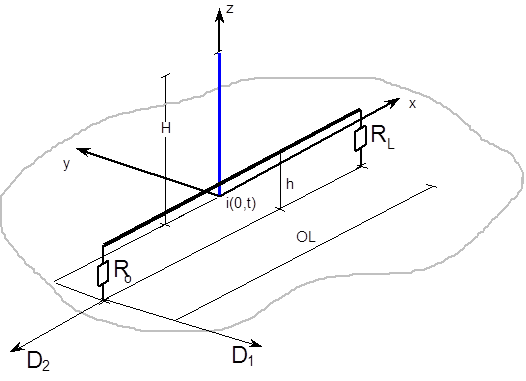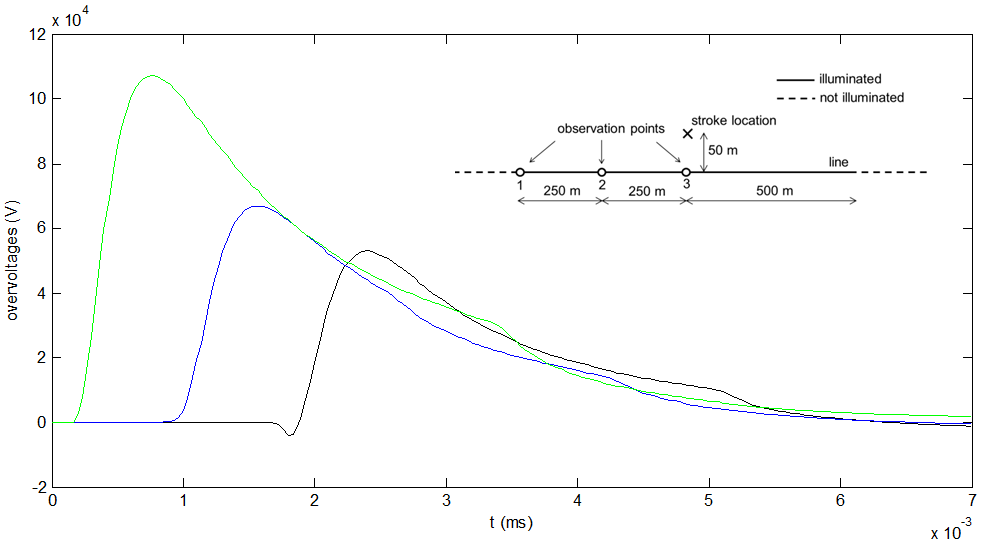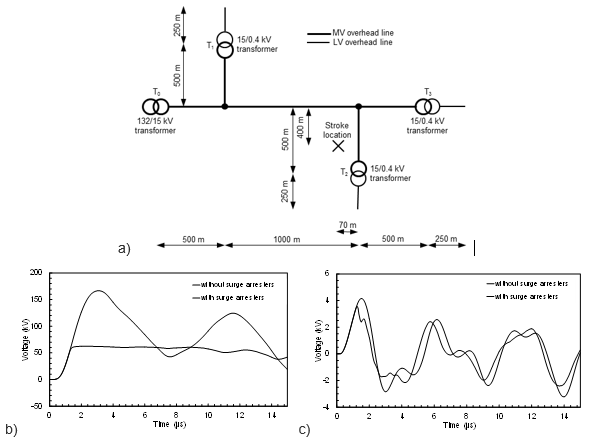# LIOV Toolbox

Simulation of lightning induced overvoltages

LIOV-EMTP® is an EMTP®module developed for the calculation of lightning-induced overvoltages on an overhead distribution network.

The LIOV (Lightning-Induced OverVoltage) code allows the calculation of lightning-induced overvoltages on multiconductor lines above a lossy soil as a function of the line geometry, lightning current waveshape and stroke location, return-stroke velocity and soil electrical parameters.Definition of the geometry for the calculation of the LEMP and its coupling with an overhead line

# 1 hour free technical call

## Get an introduction to the LIOV Toolbox with EMTP®

In order to deal with distribution networks having complex, realistic topology and configuration, the LIOV code has been interfaced with the Electromagnetic Transient Program (EMTP®).

The LIOV-EMTP® code is software developed by Alma Mater Studiorum - University of Bologna (Italy), Ecole Polytechnique Fédérale de Lausanne - EPFL (Switzerland), with the support of University of Rome “La Sapienza” and of Centro Elettrotecnico Sperimentale Italiano “G. Motta” CESI S.p.A. (Italy).

The evaluation of lightning induced overvoltages is performed in the following way:

• The lightning return-stroke electromagnetic field change is calculated along the line employing a lightning return-stroke model, namely a model that describes the spatial and temporal distribution of the return stroke current along the channel. To this end, the return stroke channel is considered as a straight, vertical antenna.

• The evaluated electromagnetic fields are used to calculate the induced overvoltages making use of a field-to-transmission line coupling model which describes the interaction between the LEMP and the line conductors. For the evaluation of the electromagnetic coupling, the implemented model is based on the transmission line theory and on the field-to-transmission line coupling model of Agrawal et al . The coupling equations are solved using the finite difference-time domain-technique (FDTD).

• For the calculation of the vertical component of the electric field, the assumption of a perfectly conducting ground is considered (this assumption has been shown to be reasonable for distances not exceeding a few kilometers or so). On the other hand, the horizontal component of the electric field, which is appreciably affected by the finite conductivity of the ground, is computed using the Cooray-Rubinstein formula [5, 6].

The LIOV code has been experimentally validated by means of several experimental data, related to natural and triggered lightning experiments, and by means of Nuclear Electromagnetic Pulse Simulators and reduced scale models .Overvoltages on a 10-m high medium voltage line above a 10 mS/m conductiing soil due to a typical subsequent return stroke (12 kA peak).

The analysis of more complex system configurations composed by several lines and power components can be dealt with by using the LIOV-EMTP® module based on the interfacing method presented in . An example of a system that can be simulated with the LIOV-EMTP® module is shown below, taken from .Comparison between the overvoltages calculated at the terminals of a 100 kVA transformer T2 due to a typical first return stroke (31 kA peak):  a) topology of the distribution network; b) MV side; c) LV side.

References:

 C. A. Nucci and F. Rachidi, “Interaction of electromagnetic fields generated by lightning with overhead electrical networks,” in The Lightning Flash, 2nd ed., V. Cooray, Ed. London: The IET, 2014, pp. 559–610.

 C. A. Nucci, C. Mazzetti, F. Rachidi, and M. Ianoz, “On lightning return stroke models for LEMP calculations,” in Proceedings of 19th International Conference on Lightning Protection, Graz, Austria, 1988, pp. 464–470.

 F. Napolitano, “An analytical formulation of the electromagnetic field generated by lightning return strokes,” IEEE Trans. Electromagn. Compat., vol. 53, no. 1, pp. 108–113, Feb. 2011.

 A. K. Agrawal, H. J. Price, and S. H. Gurbaxani, “Transient response of multiconductor transmission lines excited by a nonuniform electromagnetic field,” IEEE Trans. Electromagn. Compat., vol. 22, no. 2, pp. 119–129, May 1980.

 V. Cooray, “Horizontal fields generated by return strokes,” Radio Sci., vol. 27, no. 4, pp. 529–537, Jul. 1992.

 M. Rubinstein, “An approximate formula for the calculation of the horizontal electric field from lightning at close, intermediate, and long range,” IEEE Trans. Electromagn. Compat., vol. 38, no. 3, pp. 531–535, Aug. 1996.

 A. Borghetti, J. A. Gutierrez, C. A. Nucci, M. Paolone, E. Petrache, and F. Rachidi, “Lightning-induced voltages on complex distribution systems: models, advanced software tools and experimental validation,” J. Electrostat., vol. 60, no. 2–4, pp. 163–174, 2004.

 M. Paolone, F. Rachidi, A. Borghetti, C. A. Nucci, M. Rubinstein, V. A. Rakov, and M. A. Uman, “Lightning electromagnetic field coupling to overhead lines: theory, numerical simulations, and experimental validation,” IEEE Trans. Electromagn. Compat., vol. 51, no. 3, pp. 532–547, Aug. 2009.

 F. Napolitano, A. Borghetti, C. A. Nucci, M. Paolone, F. Rachidi, and J. Mahseredjian, “An advanced interface between the LIOV code and the EMTP®,” in Proceedings of 29th International Conference on Lightning Protection, Uppsala, Sweden, 2008.

 A. Borghetti, A. S. Morched, F. Napolitano, C. A. Nucci, and M. Paolone, “Lightning-induced overvoltages transferred through distribution power transformers,” IEEE Trans. Power Deliv., vol. 24, no. 1, pp. 360–372, Jan. 2009.

X